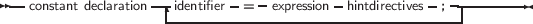### 2.1 Ordinary constants

Ordinary constants declarations are constructed using an identifier name followed by an “=” token, and followed by an optional expression consisting of legal combinations of numbers, characters, boolean values or enumerated values as appropriate. The following syntax diagram shows how to construct a legal declaration of an ordinary constant.

_________________________________________________________________________________________________________
Constant declaration___________________________________________________________________

The compiler must be able to evaluate the expression in a constant declaration at compile time. This means that most of the functions in the Run-Time library cannot be used in a constant declaration. Operators such as +, -, *, /, not, and, or, div, mod, ord, chr, sizeof, pi, int, trunc, round, frac, odd can be used, however. For more information on expressions, see chapter 12, page 583.

When a previously declared ordinary constant is used in the code, the compiler will insert the actual value of the constant instead of the constant name. That is, the following two pieces of code are entirely equivalent:

Const
One = 1;

begin
Writeln(One);
end.

The above will produce the same code as if one had written:

begin
Writeln(1);
end.

Only constants of the following types can be declared:

• Ordinal types
• Set types
• Pointer types (but the only allowed value is Nil).
• Real types
• Char,
• String

The following are all valid constant declarations:

Const
e = 2.7182818;  { Real type constant. }
a = 2;          { Ordinal (Integer) type constant. }
c = '4';        { Character type constant. }
s = 'This is a constant string'; {String type constant.}
sc = chr(32)
ls = SizeOf(Longint);
P = Nil;
Ss = [1,2];

Assigning a value to an ordinary constant is not permitted. Thus, given the previous declaration, the following will result in a compiler error:

s := 'some other string';

For string constants, the type of the string is dependent on some compiler switches. If a specific type is desired, a typed constant should be used, as explained in the following section.

Prior to version 1.9, Free Pascal did not correctly support 64-bit constants. As of version 1.9, 64-bit constants can be specified.